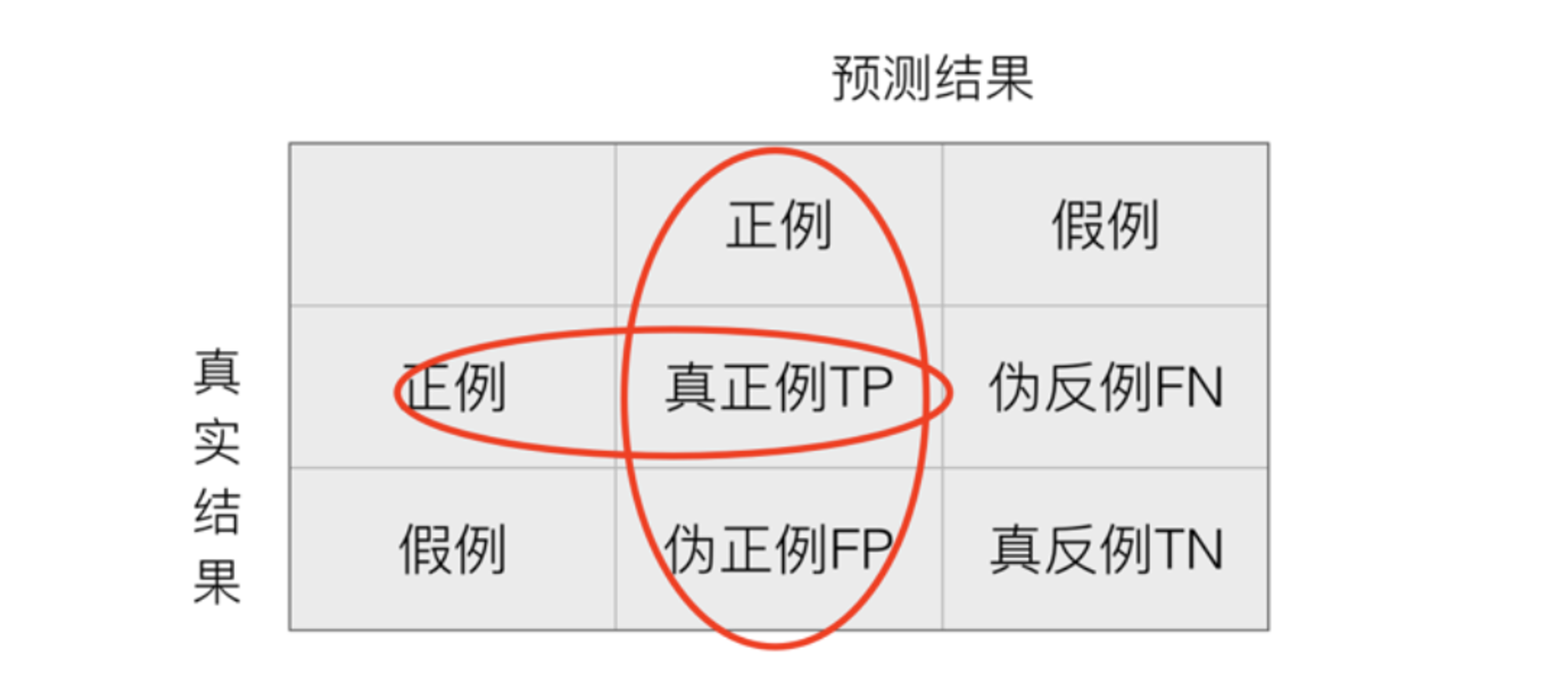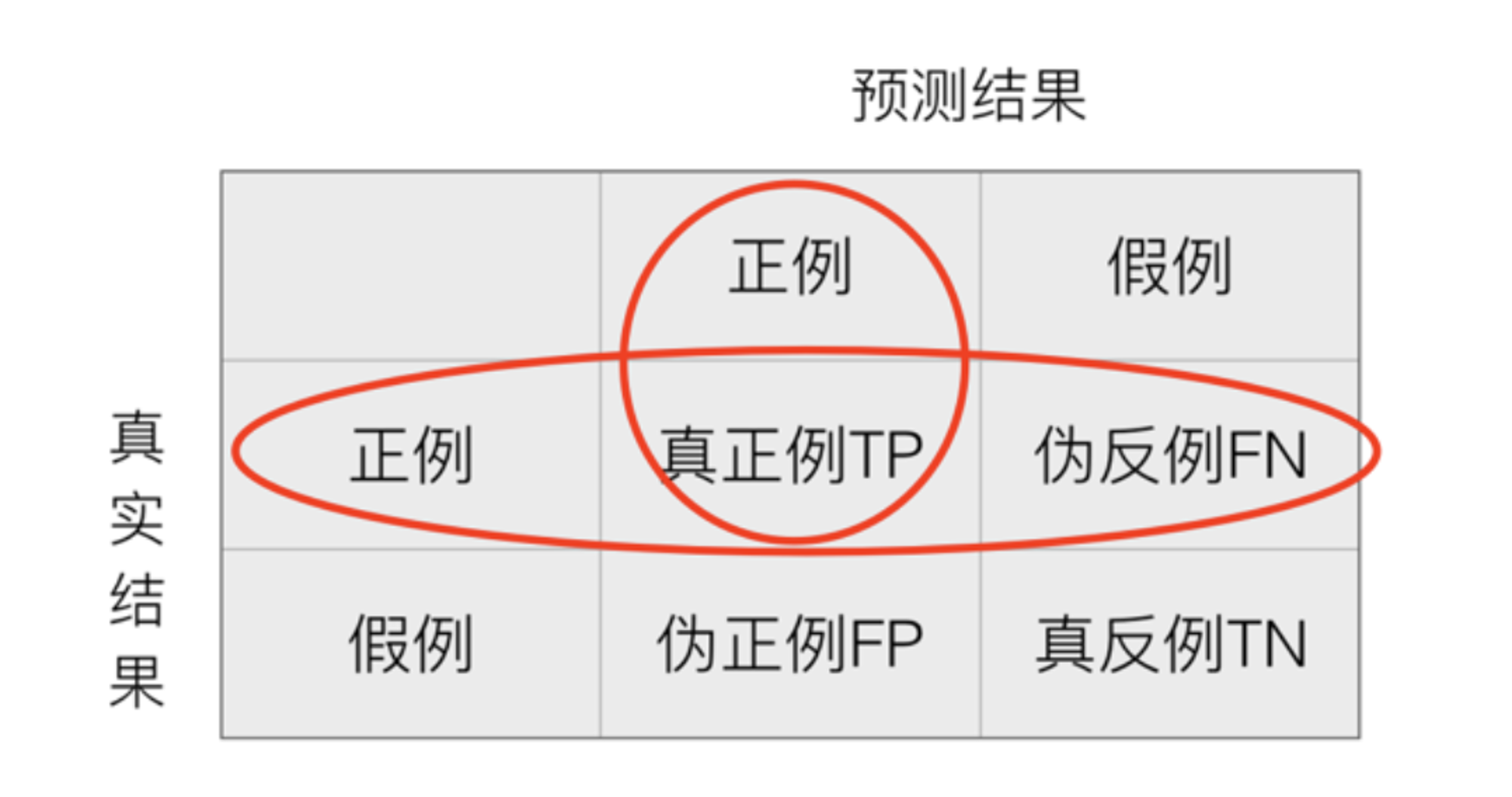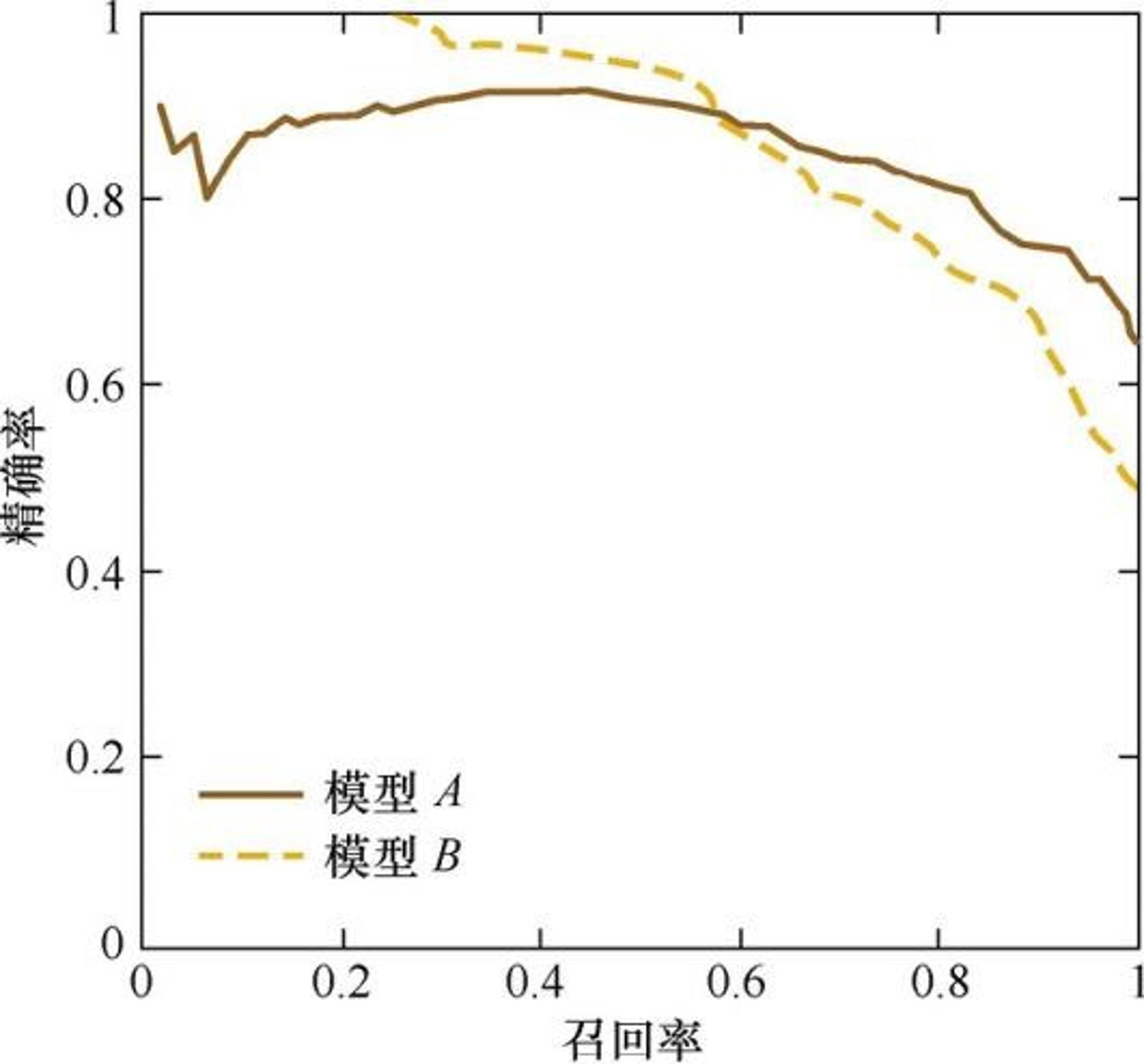墨滴lymboy

2021/06/18  阅读：47  主题：草原绿

# 基于sklearn的分类模型评估方法F1 score

F1 score是精确率和召回率的调和平均值

P-R曲线

P-R曲线的横轴是召回率，纵轴是精确率。对于一个排序模型来说，其P-R曲线上的一个点代表着，在某一阈值下，模型将大于该阈值的结果判定为正样本， 小于该阈值的结果判定为负样本，此时返回结果对应的召回率和精确率。整条P-R 曲线是通过将阈值从高到低移动而生成的。RMSE

ROC曲线

ROC曲线的横坐标为假阳性率（False Positive Rate，FPR）；纵坐标为真阳性 率（True Positive Rate，TPR）。FPR和TPR的计算方法分别为

ROC曲线是通过不断移动分类器的“截断点”来生成曲线上的一组关 键点的，通过动态地调整截断点，从最高的得分开始（实际上是从正无穷开始，对应 着ROC曲线的零点），逐渐调整到最低得分，每一个截断点都会对应一个FPR和 TPR，在ROC图上绘制出每个截断点对应的位置，再连接所有点就得到最终的 ROC曲线。

AUC

AUC指的是ROC曲线下的面积大小，该值能够量化地反映基于 ROC曲线衡量出的模型性能。计算AUC值只需要沿着ROC横轴做积分就可以了。 由于ROC曲线一般都处于 这条直线的上方（如果不是的话，只要把模型预测的 概率反转成1−p就可以得到一个更好的分类器），所以AUC的取值一般在0.5～1之 间。AUC越大，说明分类器越可能把真正的正样本排在前面，分类性能越好。

• 加载数据集
## Load Data and train model# 对于本示例，我们从 OpenML <https://www.openml.org/d/1464> 加载输血服务中心数据集。这是一个二元分类问题，目标是个人是否献血。然后将数据拆分为训练数据集和测试数据集，并使用训练数据集拟合逻辑回归。from sklearn.datasets import fetch_openmlfrom sklearn.preprocessing import StandardScalerfrom sklearn.pipeline import make_pipelinefrom sklearn.linear_model import LogisticRegressionfrom sklearn.model_selection import train_test_splitX, y = fetch_openml(data_id=1464, return_X_y=True)X_train, X_test, y_train, y_test = train_test_split(X, y, stratify=y)clf = make_pipeline(StandardScaler(), LogisticRegression(random_state=0))clf.fit(X_train, y_train)
• 展示混淆矩阵
# 使用拟合模型，我们计算模型对测试数据集的预测。这些预测用于计算使用 :class:ConfusionMatrixDisplay 绘制的混淆矩阵from sklearn.metrics import confusion_matrixfrom sklearn.metrics import ConfusionMatrixDisplayy_pred = clf.predict(X_test)cm = confusion_matrix(y_test, y_pred)cm_display = ConfusionMatrixDisplay(cm).plot()
• 绘制ROC曲线
# roc 曲线需要来自估计器的概率或非阈值决策值。由于逻辑回归提供了一个决策函数，我们将使用它来绘制 roc 曲线：from sklearn.metrics import roc_curvefrom sklearn.metrics import RocCurveDisplayy_score = clf.decision_function(X_test)fpr, tpr, _ = roc_curve(y_test, y_score, pos_label=clf.classes_)roc_display = RocCurveDisplay(fpr=fpr, tpr=tpr).plot()
• 绘制P-R曲线
from sklearn.metrics import precision_recall_curvefrom sklearn.metrics import PrecisionRecallDisplayprec, recall, _ = precision_recall_curve(y_test, y_score,                                         pos_label=clf.classes_)pr_display = PrecisionRecallDisplay(precision=prec, recall=recall).plot()
• 联合展示
import matplotlib.pyplot as pltfig, (ax1, ax2) = plt.subplots(1, 2, figsize=(12, 8))roc_display.plot(ax=ax1)pr_display.plot(ax=ax2)plt.show()

• sklearn中提供了非常多的算法实现和示例代码，用户只要查阅相关API即可，可节约时间
• 其实还有很多的模型评估方法，例如西瓜书中还提到了代价敏感错误率与代价曲线，有兴趣的读者可进一步了解

### 参考资料

• https://scikit-learn.org/stable/modules/generated/sklearn.datasets.make_classification.html#sklearn.datasets.make_classification
• https://zhuanlan.zhihu.com/p/31256633
• https://www.cnblogs.com/xiximayou/p/13180579.html
• https://vel.life/机器学习进阶/百面机器学习.pdf
• https://blog.csdn.net/qq_44642370/article/details/112290641
• https://github.com/Sophia-11/Machine-Learning-Notes
• https://datawhalechina.github.io/pumpkin-booklymboy

2021/06/18  阅读：47  主题：草原绿